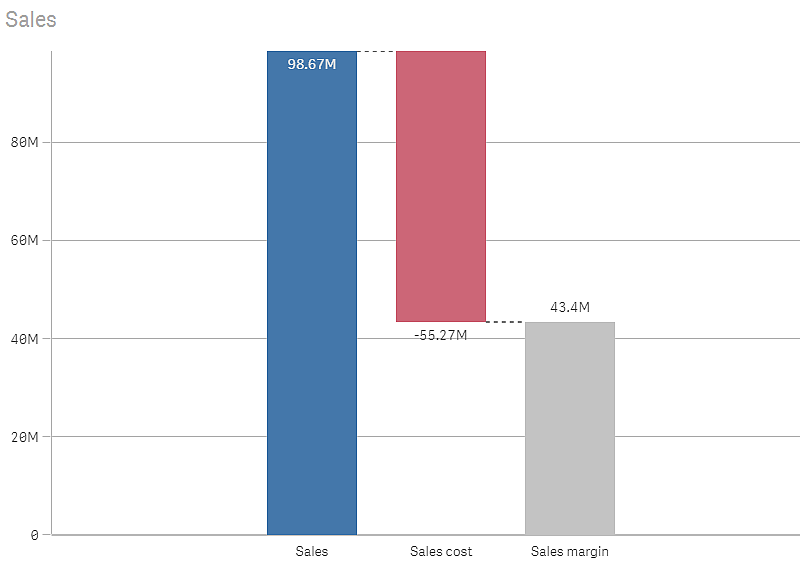# Waterfall chart

The waterfall chart is suitable for illustrating how an initial value is affected by intermediate positive and negative values. The starting and the final values are represented by whole bars, and intermediate values by floating bars. You can also show subtotals in the chart.

Waterfall chart displaying Sales, Sales cost and Sales margin.## When to use it

The waterfall chart is suitable for illustrating how an initial value is affected by intermediate positive and negative values. One example of this is an income statement, when you want to show the positive and negative contributions of different accounts.

The waterfall chart provides a quick understanding of the transition of a value.

The waterfall chart is not relevant for detailed analysis of the data as you can't make selections in the chart or expand the data.

## Creating a waterfall chart

You can create a waterfall chart on the sheet you are editing.

In a waterfall chart you need to use one measure for each bar in the chart. The order of the measures defines the order of the bars in the chart. For each measure, you need to define how it affects the previous value. You can add up to 30 measures in a waterfall chart.

1. From the assets panel, drag an empty waterfall chart to the sheet.

This is the first bar of the chart. By default, it will use the measure operation Add, and show a positive value.

This is the second bar of the chart. If you want to show this measure as a negative contribution, change Measure operation to Subtract.

4. Continue to add measures, setting Measure operation to Add or Subtract depending on how you want them to contribute.

5. Add subtotals. There are two ways of adding subtotal bars to the chart:

• If you have a data field containing subtotal data, add a measure with the subtotal data and select Subtotals as Measure operation.
• If you don't have a data field containing subtotal data, you can add an automatically calculated subtotal by selecting the Subtotals check box of the measure before where you want the subtotal bar.

When you have created the waterfall chart, you may want to adjust its appearance and other settings in the properties panel.

You can use the Measure operation option of each measure to set how it affects the previous value.

The measure value adds to the previous bar. If this is the first measure, a whole bar is shown starting at 0.

• Subtract

The measure value subtracts from the previous bar.

Note: If the data already contains a negative sign, the result of subtraction will be a positive change.
• Subtotals

The measure value is considered a subtotal.

Tip: If you do not have sub-totals as a field, you can add subtotals automatically by enabling Subtotals in the measure before you want the subtotal.

In the waterfall chart shown above, the first bar, Sales, is defined as Add. The second bar, Sales cost, is defined as Subtract, and the third bar, Sales margin, is defined as Subtotals.

Related learning: